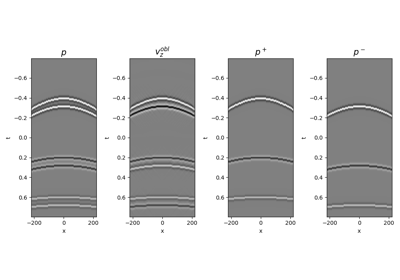# pylops.waveeqprocessing.WavefieldDecomposition¶

pylops.waveeqprocessing.WavefieldDecomposition(p, vz, nt, nr, dt, dr, rho, vel, nffts=(None, None), critical=100.0, ntaper=10, scaling=1.0, kind='inverse', restriction=None, sptransf=None, solver=<function lsqr at 0x7fc33cc3bc80>, dottest=False, dtype='complex128', **kwargs_solver)[source]

Up-down wavefield decomposition.

Apply seismic wavefield decomposition from its multi-component (pressure and vertical particle velocity) data.

Parameters: p : np.ndarray Pressure data of of size $$\lbrack n_r \times n_t \rbrack$$ (or $$\lbrack n_{r,sub} \times n_t \rbrack$$ in case a restriction operator is provided, and $$n_{r,sub}$$ must agree with the size of the output of this an operator) vz : np.ndarray Vertical particle velocity data of size $$\lbrack n_r \times n_t \rbrack$$ (or $$\lbrack n_{r,sub} \times n_t \rbrack$$) nt : int Number of samples along the time axis nr : np.ndarray Number of samples along the receiver axis of the separated pressure consituents dt : float Sampling along the time axis dr : float Sampling along the receiver array of the separated pressure consituents rho : float Density along the receiver array (must be constant) vel : float Velocity along the receiver array (must be constant) nffts : tuple, optional Number of samples along the wavenumber and frequency axes critical : float, optional Percentage of angles to retain in obliquity factor. For example, if critical=100 only angles below the critical angle $$\frac{f(k_x)}{vel}$$ will be retained ntaper : float, optional Number of samples of taper applied to obliquity factor around critical angle kind : str, optional Type of separation: inverse (default) or analytical scaling : float, optional Scaling to apply to the particle velocity data at the restriction : pylops.LinearOperator, optional Restriction operator sptransf : pylops.LinearOperator, optional Sparsifying operator solver : float, optional Function handle of solver to be used if kind='inverse' dottest : bool, optional Apply dot-test dtype : str, optional Type of elements in input array. **kwargs_solver Arbitrary keyword arguments for chosen solver pup : np.ndarray Up-going wavefield pdown : np.ndarray Down-going wavefield KeyError If kind is neither analytical nor inverse

Notes

Up- and down-going components of seismic data ($$p^-(x, t)$$ and $$p^+(x, t)$$) can be estimated from multi-component data ($$p(x, t)$$ and $$v_z(x, t)$$) by computing the following expression :

$\begin{split}\begin{bmatrix} \mathbf{p^+}(k_x, \omega) \\ \mathbf{p^-}(k_x, \omega) \end{bmatrix} = \frac{1}{2} \begin{bmatrix} 1 & \frac{\omega \rho}{k_z} \\ 1 & - \frac{\omega \rho}{k_z} \\ \end{bmatrix} \begin{bmatrix} \mathbf{p}(k_x, \omega) \\ \mathbf{v_z}(k_x, \omega) \end{bmatrix}\end{split}$

if kind='analytical' or alternatively by solving the equation in ptcpy.waveeqprocessing.UpDownComposition2D as an inverse problem, if kind='inverse'.

The latter approach has several advantages as data regularization can be included as part of the separation process allowing the input data to be aliased. This is obtained by solving the following problem:

$\begin{split}\begin{bmatrix} \mathbf{p} \\ s*\mathbf{v_z} \end{bmatrix} = \begin{bmatrix} \mathbf{R}\mathbf{F} & 0 \\ 0 & s*\mathbf{R}\mathbf{F} \end{bmatrix} \mathbf{W} \begin{bmatrix} \mathbf{F}^H \mathbf{S} & 0 \\ 0 & \mathbf{F}^H \mathbf{S} \end{bmatrix} \mathbf{p^{\pm}}\end{split}$

where $$\mathbf{R}$$ is a ptcpy.basicoperators.Restriction operator and $$\mathbf{S}$$ is sparsyfing transform operator (e.g., ptcpy.signalprocessing.Radon2D).

  Wapenaar, K. “Reciprocity properties of one-way propagators”, Geophysics, vol. 63, pp. 1795-1798. 1998.

## Examples using pylops.waveeqprocessing.WavefieldDecomposition¶12. Seismic wavefield decomposition